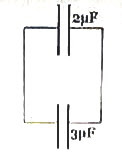## Sunday, February 17, 2008

### Two Questions (MCQ) Involving Electric Power

(1) A current I ampere gets divided and flows into a parallel network of resistors as shown in the adjoining figure. If the power dissipated in the 1 Ω resistor is P watt, what is the total power (in watt) dissipated in the branch containing the two 3 Ω resistors?

(a) P

(b) 6P

(c) 3P

(d) P/2

(e) 3P/2

If the current through the 1 Ω resistor is I1, the current through the branch containing the two 3 Ω resistors is I1/2.

[Note that the P.D across the three branches is the same and hence the current through the 6 Ω branch must be half the current through the 3 Ω branch].

The power dissipated in the 1 Ω resistor is

I12 ×1= P

Therefore, the power dissipated in the branch containing the two 3 Ω resistors is

(I1/2) 2×6 = 6P/4 = 3P/2 watt.

(2) A battery of internal resistance R ohm and output V volt is connected across a variable resistor. The heat generated in the variable resistor is maximum when the current in it is

(a) V/2R

(b) V/4R

(c) 4V/R

(d) V/R

(e) None of the above

You may be remembering the maximum power transfer theorem which states that a direct current source will transfer maximum power to a load when the resistance of the load is equal to the internal resistance of the source.

In the above problem, since the internal resistance of the battery is R, the power dissipated in the variable resistor is maximum when its resistance also is R. The total resistance in the circuit in this condition is R+R = 2R and the current is V/2R.

## Tuesday, February 05, 2008

### Electrostatics- Multiple Choice Questions Involving Capcitors

The following questions will help you to understand the calculation of common potential difference and the energy loss on connecting capacitors.(1) A 2 µF capacitor is charged by a 10 V battery. It is then disconnected from the battery and connected to an uncharged 3 µF capacitor (fig.). The total electrostatic energy stored by the system (of the two capacitors) is (in microjoule)

(a) 40

(b) 60

(c) 80

(d) 100

(e) 200

The charge will be strictly conserved and hence the total charge on the two capacitors will be the same as the initial charge on the 2 μF capacitor. Therefore, we have (from Q = C1 V1 = C2 V2),

2×10–6×10 = (2+3)×10–6×V , where V is the common potential difference between the plates of the capacitors on connecting together. Therefore,

V = 4 volts.

The electrostatic energy stored by the system is ½ (C1+C2)V2 = (½)×5×10–6×42 = 40 μJ

(2) In the above question, what is the difference between the initial and final electrostatic energies of the system?

(a) 40

(b) 60

(c) 80

(d) 100

(e) zero

The initial electrostatic energy in the system of the two capacitors is

(½) C1V12 = (½)×2×10–6×102 = 100 μJ

The final energy, as w have seen above, is 40 μJ. Therefore the difference between the initial and final electrostatic energies of the system is 100 – 40 = 60 μJ